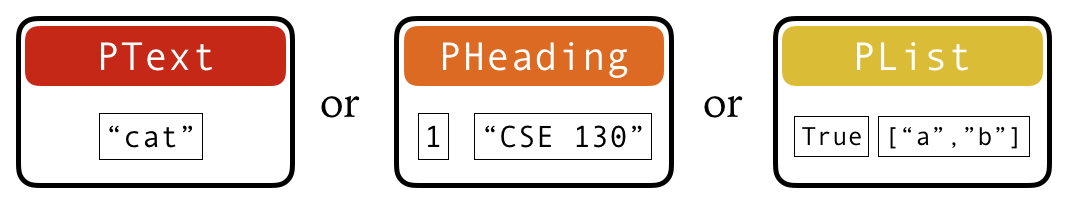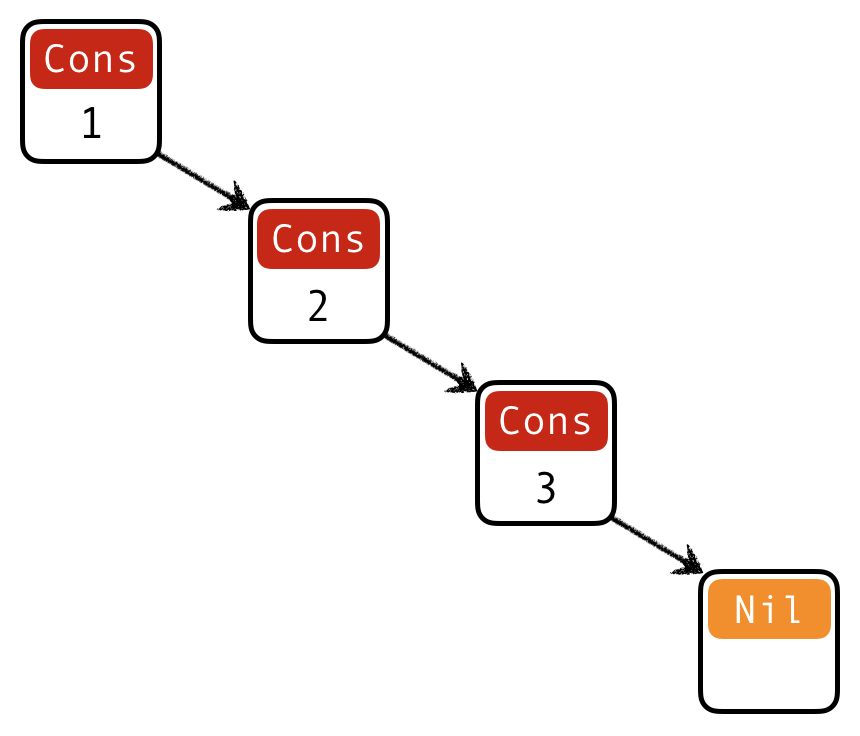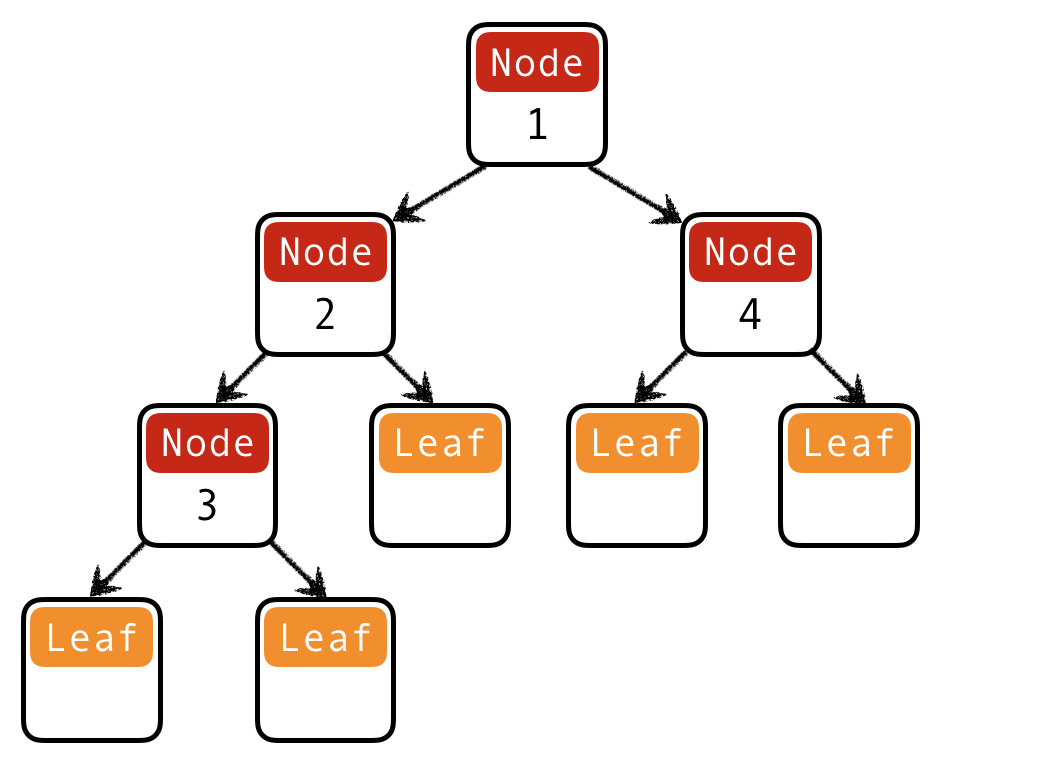# Datatypes and Recursion

## Plan for this week

Last week:

• built-in data types
• base types, tuples, lists (and strings)
• writing functions using pattern matching and recursion

This week:

• user-defined data types
• and how to manipulate them using pattern matching and recursion

## Representing complex data

We’ve seen:

• base types: `Bool`, `Int`, `Integer`, `Float`
• some ways to build up types: given types `T1, T2`

• functions: `T1 -> T2`
• tuples: `(T1, T2)`
• lists: `[T1]`

Algebraic Data Types: a single, powerful technique for building up types to represent complex data

• lets you define your own data types
• subsumes tuples and lists!

## Building data types

Three key ways to build complex types/values:

1. Product types (each-of): a value of `T` contains a value of `T1` and a value of `T2`

2. Sum types (one-of): a value of `T` contains a value of `T1` or a value of `T2`

3. Recursive types: a value of `T` contains a sub-value of the same type `T`

## Product types

Tuples can do the job but there are two problems…

``````deadlineDate :: (Int, Int, Int)

-- | Deadline date extended by one day
extendedDate :: (Int, Int, Int) -> (Int, Int, Int)
extendedDate = ...``````

Can you spot them?

A type synonym for `T`: a name that can be used interchangeably with `T`

``````type Date = (Int, Int, Int)
type Time = (Int, Int, Int)

-- | Deadline date extended by one day
extendedDate :: Date -> Date
extendedDate = ...``````

### 2. Unsafe

We want this to fail at compile time!!!

``extendedDate deadlineTime``

Solution: construct two different datatypes

``````data Date = Date Int Int Int
data Time = Time Int Int Int
-- constructor^   ^parameter types

deadlineDate = Date 4 29 2020

deadlineTime = Time 11 59 59``````

### Record syntax

Haskell’s record syntax allows you to name the constructor parameters:

``data Date = Date Int Int Int``
• you can write:

``````data Date = Date {
month :: Int,
day   :: Int,
year  :: Int
}``````
• then you can do:

``````deadlineDate = Date 4 29 2020

dealineMonth = month deadlineDate -- use field name as a function``````

## Building data types

Three key ways to build complex types/values:

1. Product types (each-of): a value of `T` contains a value of `T1` and a value of `T2` [done]

2. Sum types (one-of): a value of `T` contains a value of `T1` or a value of `T2`

3. Recursive types: a value of `T` contains a sub-value of the same type `T`

## Example: NanoMarkdown

Suppose I want to represent a text document with simple markup

Each paragraph is either:

• plain text (`String`)
• heading: level and text (`Int` and `String`)
• list: ordered? and items (`Bool` and `[String]`)

I want to store all paragraphs in a list

``````doc = [
(1, "Notes from 130")                        -- Lvl 1 heading
, "There are two types of languages:"          -- Plain text
, (True, ["those people complain about"        -- Ordered list
, "those no one uses"])
]``````

But this doesn’t type check!!!

## Sum Types

Solution: construct a new type for paragraphs that is a sum (one-of) the three options!

Each paragraph is either:

• plain text (`String`)
• heading: level and text (`Int` and `String`)
• list: ordered? and items (`Bool` and `[String]`)
``````data Paragraph =
PText String          -- 3 constructors,
| PHeading Int String   -- each with different
| PList Bool [String]   -- parameters``````

## QUIZ

``````data Paragraph =
PText String | PHeading Int String | PList Bool [String]``````

What would GHCi say to

``>:t PText "Hey there!"``

A. Syntax error

B. Type error

C. `PText`

D. `String`

E. `Paragraph`

## Constructing datatypes

``````data T =
C1 T11 .. T1k
| C2 T21 .. T2l
| ..
| Cn Tn1 .. Tnm``````

`T` is the new datatype

`C1 .. Cn` are the constructors of `T`

A value of type `T` is

• either `C1 v1 .. vk` with `vi :: T1i`
• or `C2 v1 .. vl` with `vi :: T2i`
• or
• or `Cn v1 .. vm` with `vi :: Tni`

You can think of a `T` value as a box:

• either a box labeled `C1` with values of types `T11 .. T1k` inside
• or a box labeled `C2` with values of types `T21 .. T2l` inside
• or
• or a box labeled `Cn` with values of types `Tn1 .. Tnm` insideConstructors as boxes

## Datatype for Paragraphs

``````data Paragraph =
PText String
| PList Bool [String]``````Paragraph Constructors

Apply a constructor = pack some values into a box (and label it)

• `PText "cat"`
• put `"cat"` in a box labeled `PText`
• `PHeading 1 "CSE 130"`
• put `1` and `"CSE 130"` in a box labeled `PHeading`
• Boxes have different labels but same type (`Paragraph`)Paragraph Values

## QUIZ

``````data Paragraph =
PText String | PHeading Int String | PList Bool [String]``````

What would GHCi say to

``>:t [PHeading 1 "Introduction", PText "Hey there!"]``

A. Syntax error

B. Type error

C. `Paragraph`

D. `[Paragraph]`

E. `[String]`

## Example: NanoMarkdown

``````data Paragraph =
PText String | PHeading Int String | PList Bool [String]``````

Now I can create a document like so:

``````doc :: [Paragraph]
doc = [
, PText "There are two types of languages:"
, PList True [ "those people complain about"
, "those no one uses"]]``````

Now I want convert documents in to HTML.

I need to write a function:

``````html :: Paragraph -> String
html p = ??? -- depends on the kind of paragraph!``````

How to tell what’s in the box?

• Look at the label!

## Pattern matching

Pattern matching = looking at the label and extracting values from the box

• we’ve seen it before
• but now for arbitrary datatypes
``````html :: Paragraph -> String
html (PText str)        = ... -- It's a plain text! Get string
html (PHeading lvl str) = ... -- It's a heading! Get level and string
html (PList ord items)  = ... -- It's a list! Get ordered and items``````

``````html :: Paragraph -> String
html (PText str) =           -- It's a plain text! Get string
unlines [open "p", str, close "p"]
html (PHeading lvl str) =    -- It's a heading! Get level and string
let htag = "h" ++ show lvl
in unwords [open htag, str, close htag]
html (PList ord items) =    -- It's a list! Get ordered and items
let
ltag = if ord then "ol" else "ul"
litems = [unwords [open "li", i, close "li"] | i <- items]
in unlines ([open ltag] ++ litems ++ [close ltag])

open t = "<" ++ t ++ ">"
close t = "</" ++ t ++ ">"  ``````

### Dangers of pattern matching (1)

``````html :: Paragraph -> String
html (PText str) = ...
html (PList ord items) = ...``````

What would GHCi say to:

``html (PHeading 1 "Introduction")``

Answer: Runtime error (no matching pattern)

### Dangers of pattern matching (2)

``````html :: Paragraph -> String
html (PText str)        = unlines [open "p", str, close "p"]
html (PHeading lvl str) = ...
html (PList ord items)  = ...``````

What would GHCi say to:

``html (PHeading 0 "Introduction")``

Answer: `PHeading 0 "Introduction"` will be matched by `PHeading lvl str`

### Dangers of pattern matching

Beware of missing and overlapped patterns

• GHC warns you about overlapped patterns
• GHC warns you about missing patterns when called with `-W` (use `:set -W` in GHCi)

## Pattern matching expression

We’ve seen: pattern matching in equations

You can also pattern-match inside your program using the `case` expression:

``````html :: Paragraph -> String
html p =
case p of
PText str -> unlines [open "p", str, close "p"]
PList ord items -> ...``````

## QUIZ

What is the type of

``````let p = PText "Hey there!"
in case p of
PText str -> str
PList ord _ -> ord     ``````

A. Syntax error

B. Type error

C. `String`

D. `Paragraph`

E. `Paragraph -> String`

Answer: B (cases have different types)

## Pattern matching expression: typing

The `case` expression

``````case e of
pattern1 -> e1
pattern2 -> e2
...
patternN -> eN``````

has type `T` if

• each `e1``eN` has type `T`
• `e` has some type `D`
• each `pattern1``patternN` is a valid pattern for `D`
• i.e. a variable or a constructor of `D` applied to other patterns

The expression `e` is called the match scrutinee

## QUIZ

What is the type of

``````let p = PText "Hey there!"
in case p of
PText _ -> 1
PList _ _ -> 3     ``````

A. Syntax error

B. Type error

C. `Paragraph`

D. `Int`

E. `Paragraph -> Int`

## Building data types

Three key ways to build complex types/values:

1. Product types (each-of): a value of `T` contains a value of `T1` and a value of `T2` [done]

• Cartesian product of two sets: v(T)=v(T1)×v(T2)
2. Sum types (one-of): a value of `T` contains a value of `T1` or a value of `T2` [done]

• Union (sum) of two sets: v(T)=v(T1)∪v(T2)
3. Recursive types: a value of `T` contains a sub-value of the same type `T`

## Recursive types

Let’s define natural numbers from scratch:

``data Nat = ???``

``data Nat = Zero | Succ Nat``

A `Nat` value is:

• either an empty box labeled `Zero`
• or a box labeled `Succ` with another `Nat` in it!

Some `Nat` values:

``````Zero                     -- 0
Succ Zero                -- 1
Succ (Succ Zero)         -- 2
Succ (Succ (Succ Zero))  -- 3
...``````

## Functions on recursive types

Recursive code mirrors recursive data

### 1. Recursive type as a parameter

``````data Nat = Zero     -- base constructor
| Succ Nat -- inductive constructor``````

Step 1: add a pattern per constructor

``````toInt :: Nat -> Int
toInt Zero     = ... -- base case
toInt (Succ n) = ... -- inductive case
-- (recursive call goes here)``````

Step 2: fill in base case:

``````toInt :: Nat -> Int
toInt Zero     = 0   -- base case
toInt (Succ n) = ... -- inductive case
-- (recursive call goes here)``````

Step 3: fill in inductive case using a recursive call:

``````toInt :: Nat -> Int
toInt Zero     = 0           -- base case
toInt (Succ n) = 1 + toInt n -- inductive case``````

## QUIZ

What does this evaluate to?

``````let foo i = if i <= 0 then Zero else Succ (foo (i - 1))
in foo 2 ``````

A. Syntax error

B. Type error

C. `2`

D. `Succ Zero`

E. `Succ (Succ Zero)`

### 2. Recursive type as a result

``````data Nat = Zero     -- base constructor
| Succ Nat -- inductive constructor

fromInt :: Int -> Nat
fromInt n
| n <= 0    = Zero                   -- base case
| otherwise = Succ (fromInt (n - 1)) -- inductive case
-- (recursive call goes here)``````

### 3. Putting the two together

``````data Nat = Zero     -- base constructor
| Succ Nat -- inductive constructor

add :: Nat -> Nat -> Nat

sub :: Nat -> Nat -> Nat
sub n m = ???``````

``````data Nat = Zero     -- base constructor
| Succ Nat -- inductive constructor

add :: Nat -> Nat -> Nat
add Zero     m = m              -- base case
add (Succ n) m = Succ (add n m) -- inductive case

sub :: Nat -> Nat -> Nat
sub n        Zero     = n       -- base case 1
sub Zero     _        = Zero    -- base case 2
sub (Succ n) (Succ m) = sub n m -- inductive case``````

Lessons learned:

• Recursive code mirrors recursive data
• With multiple arguments of a recursive type, which one should I recurse on?
• The name of the game is to pick the right inductive strategy!

## Lists

Lists aren’t built-in! They are an algebraic data type like any other:

``````data List = Nil           -- base constructor
| Cons Int List -- inductive constructor``````
• List `[1, 2, 3]` is represented as `Cons 1 (Cons 2 (Cons 3 Nil))`

• Built-in list constructors `[]` and `(:)` are just fancy syntax for `Nil` and `Cons`

Functions on lists follow the same general strategy:

``````length :: List -> Int
length Nil         = 0              -- base case
length (Cons _ xs) = 1 + length xs  -- inductive case``````

What is the right inductive strategy for appending two lists?

``````append :: List -> List -> List
append Nil ys = ys
append (Cons x xs) ys = Cons x (append xs ys)    ``````

## Trees

Lists are unary trees:Unary tree (aka list)

``data List = Nil | Cons Int List``

How do we represent binary trees?Binary tree

## QUIZ: Binary trees I

What is a Haskell datatype that can represent this binary tree?Binary tree

(A) `data Tree = Leaf | Node Int Tree`

(B) `data Tree = Leaf | Node Tree Tree`

(C) `data Tree = Leaf | Node Int Tree Tree`

(D) `data Tree = Leaf Int | Node Tree Tree`

(E) `data Tree = Leaf Int | Node Int Tree Tree`Binary tree

``````data Tree = Leaf | Node Int Tree Tree

t1234 = Node 1
(Node 2 (Node 3 Leaf Leaf) Leaf)
(Node 4 Leaf Leaf)``````

BTW, answer D is also a binary tree, but with values stored in leaves!Leaf tree

``````data Tree = Leaf Int | Node Tree Tree

t12345 = Node
(Node (Node (Leaf 1) (Leaf 2)) (Leaf 3))
(Node (Leaf 4) (Leaf 5))``````

## Functions on trees

``````height :: Tree -> Int
height Leaf = 0
height (Node _ l r) = 1 + max (height l) (height r)``````

## Example: Calculator

I want to implement an arithmetic calculator to evaluate expressions like:

• `4.0 + 2.9`
• `3.78 – 5.92`
• `(4.0 + 2.9) * (3.78 - 5.92)`

What is a Haskell datatype to represent these expressions?

``data Expr = ???``

``````data Expr = Num Float
| Sub Expr Expr
| Mul Expr Expr``````

How do we write a function to evaluate an expression?

``````eval :: Expr -> Float
eval (Num f)     = f
eval (Add e1 e2) = eval e1 + eval e2
eval (Sub e1 e2) = eval e1 - eval e2
eval (Mul e1 e2) = eval e1 * eval e2``````

## Recursion is…

Building solutions for big problems from solutions for sub-problems

• Base case: what is the simplest version of this problem and how do I solve it?
• Inductive strategy: how do I break down this problem into sub-problems?
• Inductive case: how do I solve the problem given the solutions for subproblems?

## Why use Recursion?

1. Often far simpler and cleaner than loops

• But not always…
2. Structure often forced by recursive data

3. Forces you to factor code into reusable units (recursive functions)

## Why not use Recursion?

1. Slow

2. Can cause stack overflow

## Example: factorial

``````fac :: Int -> Int
fac n
| n <= 1    = 1
| otherwise = n * fac (n - 1)``````

Lets see how `fac 4` is evaluated:

``````<fac 4>
==> <4 * <fac 3>>              -- recursively call `fact 3`
==> <4 * <3 * <fac 2>>>        --   recursively call `fact 2`
==> <4 * <3 * <2 * <fac 1>>>>  --     recursively call `fact 1`
==> <4 * <3 * <2 * 1>>>        --     multiply 2 to result
==> <4 * <3 * 2>>              --   multiply 3 to result
==> <4 * 6>                    -- multiply 4 to result
==> 24``````

Each function call `<>` allocates a frame on the call stack

• expensive
• the stack has a finite size

Can we do recursion without allocating stack frames?

## Tail Recursion

Recursive call is the top-most sub-expression in the function body

• i.e. no computations allowed on recursively returned value

• i.e. value returned by the recursive call == value returned by function

### QUIZ: Is this function tail recursive?

``````fac :: Int -> Int
fac n
| n <= 1    = 1
| otherwise = n * fac (n - 1)``````

A. Yes

B. No

## Tail recursive factorial

Let’s write a tail-recursive factorial!

``````facTR :: Int -> Int
facTR n = loop 1 n
where
loop :: Int -> Int -> Int
loop acc n
| n <= 1    = acc
| otherwise = loop (acc * n) (n - 1)``````

Lets see how `facTR` is evaluated:

``````<facTR 4>
==>    <<loop 1  4>> -- call loop 1 4
==>   <<<loop 4  3>>> -- rec call loop 4 3
==>  <<<<loop 12 2>>>> -- rec call loop 12 2
==> <<<<<loop 24 1>>>>> -- rec call loop 24 1
==> 24                  -- return result 24! ``````

Each recursive call directly returns the result

• without further computation

• no need to remember what to do next!

• no need to store the “empty” stack frames!

## Why care about Tail Recursion?

Because the compiler can transform it into a fast loop

``````facTR n = loop 1 n
where
loop acc n
| n <= 1    = acc
| otherwise = loop (acc * n) (n - 1)``````

``````function facTR(n){
var acc = 1;
while (true) {
if (n <= 1) { return acc ; }
else        { acc = acc * n; n = n - 1; }
}
}``````
• Tail recursive calls can be optimized as a loop

• no stack frames needed!
• Part of the language specification of most functional languages

• compiler guarantees to optimize tail calls

That’s all folks!